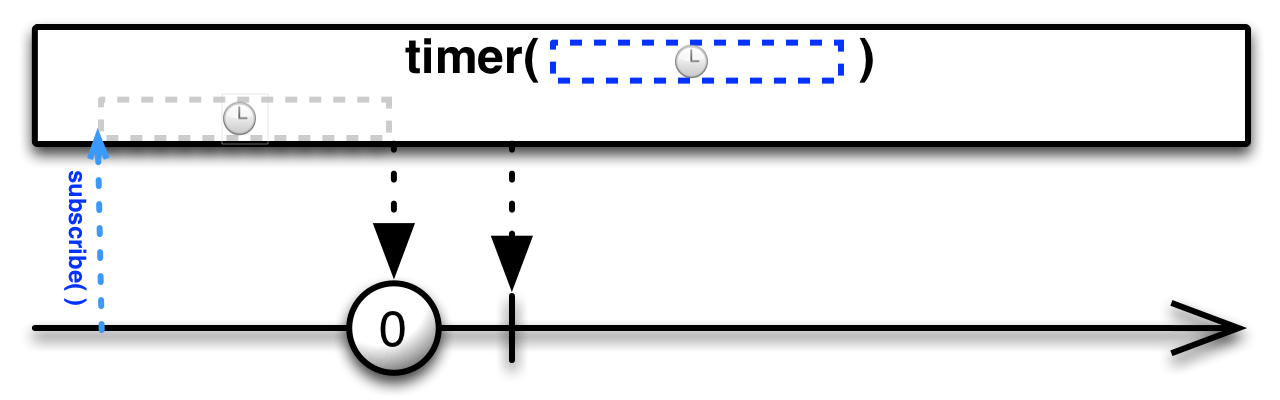# Timer¶

• Official ReactiveX documentation: Timer
classmethod Observable.timer(duetime, period=None, scheduler=None)

Returns an observable sequence that produces a value after duetime has elapsed and then after each period.

Examples:

from datetime import datetime

res = Observable.timer(datetime.now())
res = Observable.timer(datetime.now(), 1000)
res = Observable.timer(datetime.now(), Scheduler.timeout)
res = Observable.timer(datetime.now(), 1000, Scheduler.timeout)

res = Observable.timer(5000)
res = Observable.timer(5000, 1000)
res = Observable.timer(5000, scheduler=Scheduler.timeout)
res = Observable.timer(5000, 1000, Scheduler.timeout)

Parameters: Keyword Arguments: duetime – Absolute (specified as a datetime.datetime() object) or relative time (specified as an integer denoting milliseconds) at which to produce the first value. period (int) – Period to produce subsequent values (specified as an integer denoting milliseconds), or the scheduler to run the timer on. If not specified, the resulting timer is not recurring. scheduler (Scheduler) – Scheduler to run the timer on. If not specified, the timeout scheduler is used. An observable sequence that produces a value after due time has elapsed and then each period.